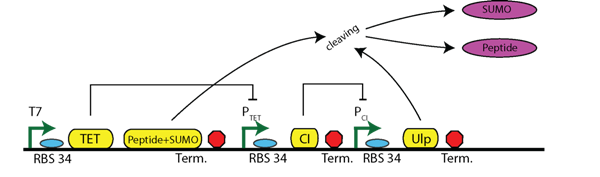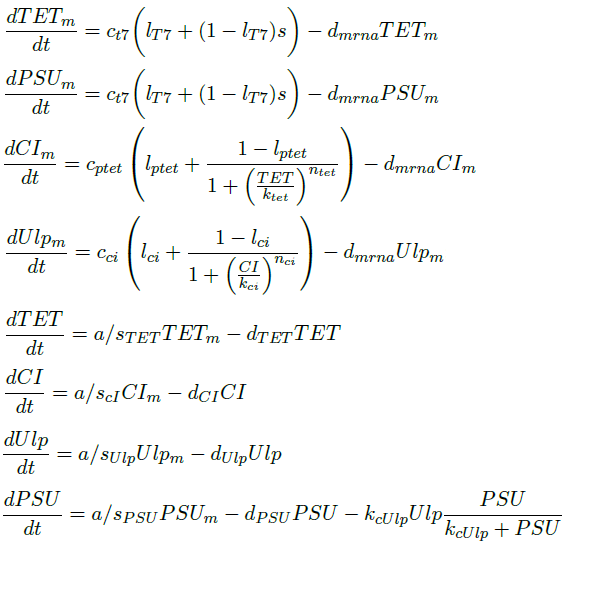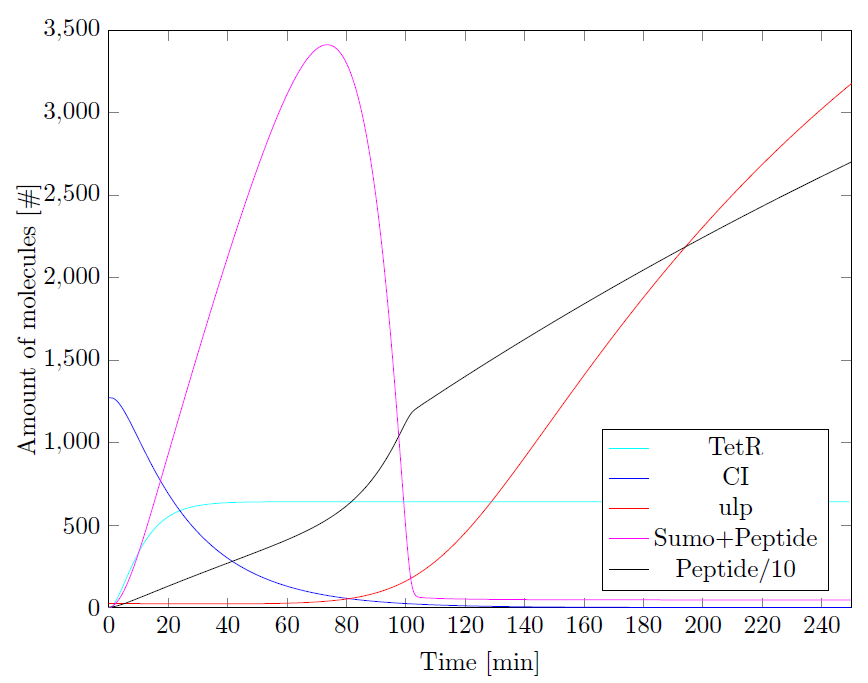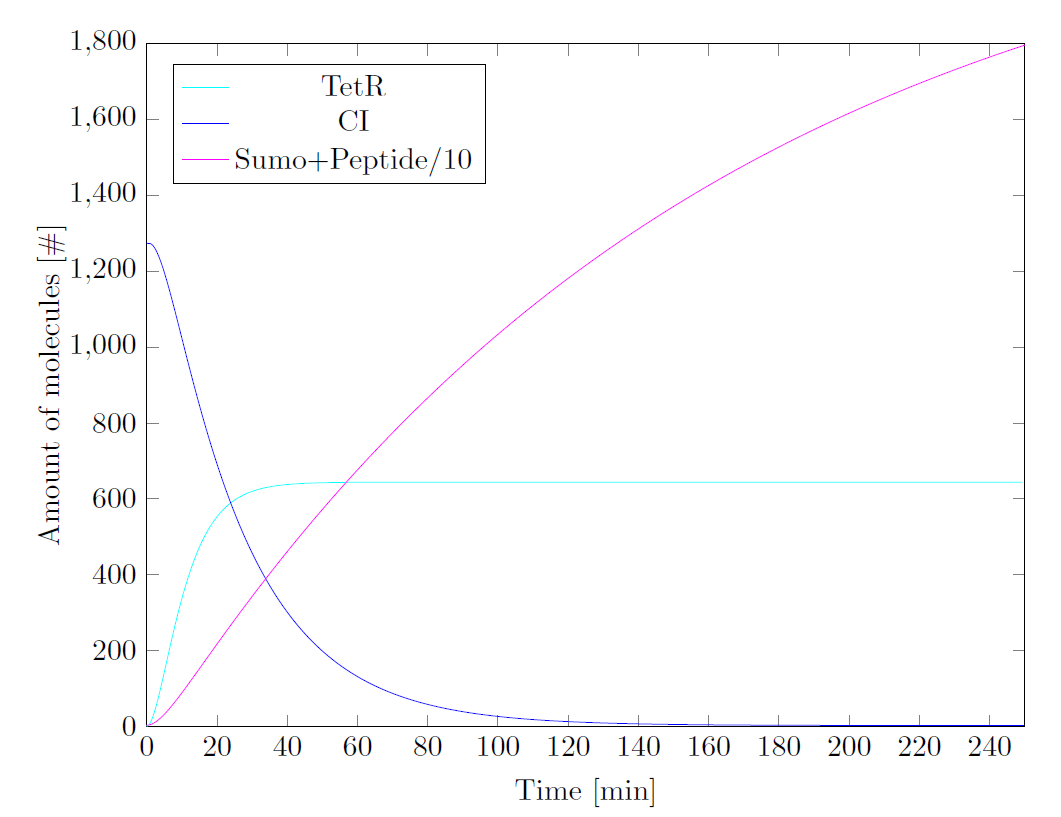# Team:TU-Delft/Timer Plus Sumo

(Difference between revisions)
 Revision as of 07:51, 17 September 2013 (view source)← Older edit Revision as of 07:52, 17 September 2013 (view source)Newer edit → Line 207: Line 207:

- In Figure 3 the circuit is modeled without Ulp present, so the SUMO is not cleaved off. This is done to be able to found experimental data. In [], an expression of a SUMO fusion protein is done with the T7 promoter. These results were 0.5 &uu;g is produced in 2L of culture. Calculating this back using an OD of 1, this results in + In Figure 3 the circuit is modeled without Ulp present, so the SUMO is not cleaved off. This is done to be able to found experimental data. In [], an expression of a SUMO fusion protein is done with the T7 promoter. These results were 0.5 μ is produced in 2L of culture. Calculating this back using an OD of 1, this results in

## Revision as of 07:52, 17 September 2013## Timer Plus Sumo

In this section the system of Figure 1 is modeled. The structure of the timer has two repressing promoters (PcI and Ptet) and the input is the T7 promoter and the output is the protease Ulp-1. This Ulp-1 cleaves off the SUMO from the produced SUMO-peptide.Figure 1: Circuit of the timer including sumo cleaving

## Differential Equations

The above circuit can be represented by the following differential equations. We assume a binary behavior of the T7 promoter. In the presence of IPTG, the T7 promoter will be active. So, we make the assumption that the T7 is binary variable with two possible states: either active 1 or inactive 0.## Parameters

For the stated parameters of the differential equations many values can be found in the literature. The degradation rates are relatively unknown, while the transcription rates vary widely. Many of these transcription rates resulted in our model in infeasible results, e.g. concentrations of millions of molecules per cell. For the PT7 a relative low transcription rate was thus selected in order to get the values in a reasonable range. The other promoters in the model we found as a fraction of the transcription rate of PT7 in the literature.

 Parameter Value Description Units Reference ca 1020 Translation rate per amino acid min-1#a-1  cT7 4.16 Maximum transcription rate of T7 #m/min  cptet 2.79 Maximum transcription rate of Ptet #m/min  cci 1.79 Maximum transcription rate of Pci #m/min  dmRNA 0.231 Degradation rate of mRNA min-1  dTET 0.1386 Degradation rate of TET min-1  dCI 0.042 Degradation rate of CI min-1  dPEP 2.1*10-3 Degradation rate of the peptide min-1 Assumed three times slower same as GFP dPSU 6.3*10-3 Degradation rate of the peptide plus SUMO min-1 Assumed the same as GFP dUlp 1.263*10-2 Degradation rate of Ulp min-1 Assumed twice the rate of GFP lt7 0.002 Leakage factor of T7 - Assumption lptet 0.002 Leakage factor of Ptet - Assumption lci 0.002 Leakage factor of Pci - Assumption ktet 6 Dissociation constant of Ptet #m  kci 20 Dissociation constant of Pci #m  kcUlp 3 Turnover rate of Ulp min-1  nci 3 Hills coefficient -  ntet 3 Hills coefficient -  s 0 or 1 Activation/Inactivation of T7 promoter Binary Assumption sci 228 Length of CI amino acids  sPSU 18 + 110 Length of peptide plus SUMO amino acids  sTET 206 Length of TET amino acids  sUlp 233 Length of Ulp1 amino acids 

## Results

Here the results are given of the simulation upon activating the T7 promoter. For starting conditions the steady state values of the concentrations are used when T7 is switched off.Figure 2: Simulation Results

A second simulation is run, in which only SUMO-peptide is produced as there is no Ulp. This will allow some confirmation of the values with the literature.Figure 3: Simulation Results without Ulp

## Discussion

In the graph of Figure 2 the effect of the timer is clearly observed, at first the peptide+SUMO is produced, and after approximatly 80 minutes more Ulp is produced leading to an increased cleaving of the SUMO. Also note that there is cleaving of the SUMO already from the start, as the promoters are somewhat leaky.

In Figure 3 the circuit is modeled without Ulp present, so the SUMO is not cleaved off. This is done to be able to found experimental data. In [], an expression of a SUMO fusion protein is done with the T7 promoter. These results were 0.5 μ is produced in 2L of culture. Calculating this back using an OD of 1, this results in